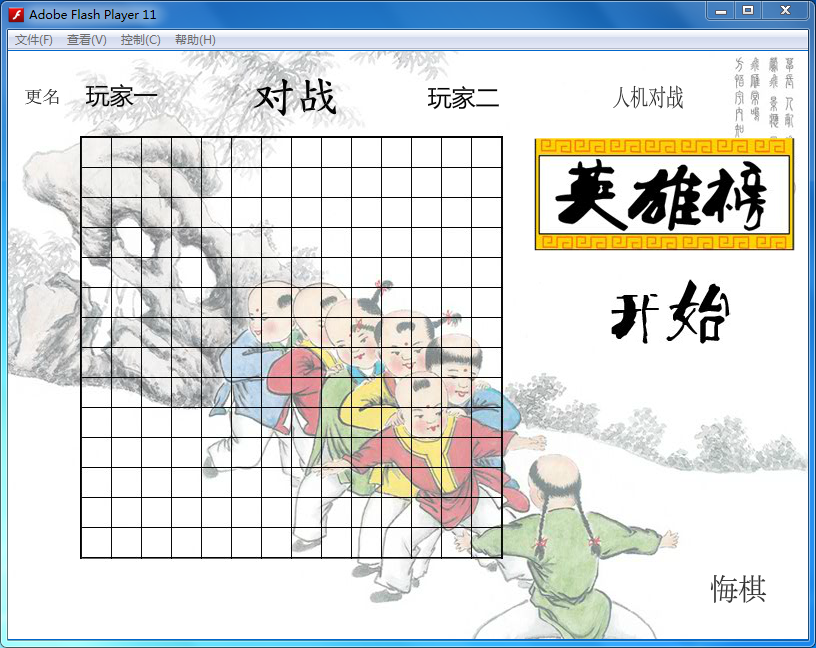flash as3.0 五子棋的游戏制作1：素材的导入

2:“开始”，“结束”按钮的制作
function start_mcclick(e:MouseEvent)
{
start_mc.visible=false;
end_mc.visible=true;
blackground.visible=false;
var i,j:int;
for(i=0;i<15;i++){
for(j=0;j<15;j++){
}
}
zhan_mc.visible=false;//将对战的影片剪辑不可视
blackwin_mc.visible=false;//将黑色赢的影片剪辑不可视
whitewin_mc.visible=false; //将白色赢的影片剪辑不可视
}
3：棋子的下落实现

var x1:int,y1:int,n:int;
x1=(event.stageX-65)/30;
y1=(event.stageY-75)/30;
{
var qia:MovieClip=new MovieClip;
{
qia=new black();
}
else//白色走棋
{
qia=new white();
}
qia.x=73+(30*(x1));
qia.y=85+(30*(y1));
aChessman.push(qia);
}

4：输赢的判断

（00）（01）（02）（03）
（10）（11）（12）（13）
（20）（21）（22）（23）
（30）（31）（32）（33）

function checkwinner(xp:int,yp:int,side:int)//胜负检查函数
{
var i:int =0;
var j:int =0;
var n:int =0;
var c:int =0;
var winner:int = 0;
//x方向
for(i=0;i<15;i++)
{
{
n++;
if(n==5){
winner=side;break;
}
}
else {n=0;}
}

//y
for(i=0;i<15;i++)
{
{
n++;
if(n==5){
winner=side;break;
}
}
else {n=0;}
}
//斜方向
n=0;
for(c=9;c>=0;c--)
{
for(i=0,j=c;j<15;i++,j++)
{
{
n++;
if(n==5){
winner=side;
break;
}
}
else {n=0;}
}
}
n=0;
for(c=9;c>0;c--)
{
for(i=c,j=0;i<15;i++,j++)
{
{
n++;
if(n==5){
winner=side;
break;
}
}
else {n=0;}
}
}
//右斜上方向
n=0;
for(c=4;c<15;c++)
{
for(i=0,j=c;j>=0;i++,j--)
{
{
n++;
if(n==5){
winner=side;
break;
}
}
else {n=0;}
}
}
n=0;
for(c=9;c>0;c--)
{
for(i=c,j=14;i<15;i++,j--)
{
{
n++;
if(n==5){
winner=side;
break;
}
}
else {n=0;}
}
}
if(winner==1){
trace("黑方胜利");
blackwin_mc.visible=true;
blackground.visible=true;
score1++;

}
if(winner==2){
trace("白色胜利");
whitewin_mc.visible=true;
blackground.visible=true;